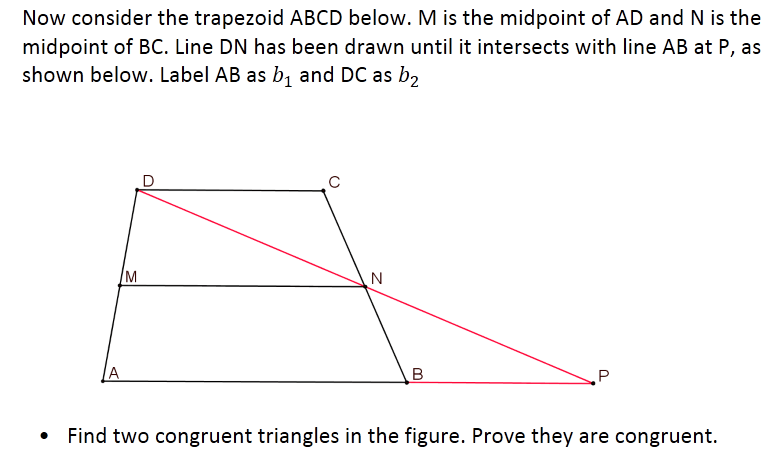# Trapezoid Formula Jigsaw

Today in class I tried a new activity I made. I had a lot of goals for this activity:

1. Show that triangle midsegments are parallel to the non-touching side and are half the length of the non-touching side.
2. Show that trapezoid midsegments/medians (I hate that my book calls them medians!) are parallel to both bases and have a length equal to the average of the bases
3. Derive the area formula of a trapezoid
4. Understand multiple justifications for the area of a trapezoid

This last is something I’ve been thinking about more and more as important in mathematical reasoning, and geometry by extension (since that’s where the US HS curriculum puts the most emphasis on formal reasoning/proof). We talk about “the proof” a lot or “where the formula comes from” but it’s important and useful for students to realize that there are often lots of ways to skin a cat, even when it comes to formal proofs.

The activity starts out simple:

• I define the midsegment of a triangle, then ask them to draw one (any one) on each triangle below• Next, I have them prove the small triangle they just created is similar to the old one. They have to notice they have two half-size segments, so they have SAS~ with a scale factor of 1/2.
• Finally, they use the similarity to show that the midsegment is half the length of and parallel to the other side.
• We just did similarity and similarity proofs, so this went pretty swimmingly, though the congruent Corresponding Angles -> parallel lines piece needed a fair bit of prompting in my class and took some time to justify.
• At this point I brought the class together to officially define and clarify the triangle midsegment theorem. Next, I give students this diagram and question.• This was tricky; the first step, identifying which triangles could be congruent, was the hardest part for them. Only one group noticed that, visually, MND was not an obtuse triangle and the other two were; many groups tried to prove MND=BPN at first! In the future I will have them trace one of the triangles they think is congruent on patty paper, and check their congruence before they embark on the proof! I also had to prompt most groups to look for parallel lines and AIAs before they could complete this.
• Which segment is congruent to DN? Which segment is congruent to DC? Mark these congruences using tick marks.
• In terms of AB and DC (the bases of the original trapezoid!), how long is the base of triangle ADP?
• What does the triangle midsegment theorem tell us about the length of MN compared to AP? What is the length of MN in terms of h, b1, and b2?
• Draw the height of the triangle corresponding to base AP (which is also the height of the original trapezoid). Label its length “h”. If the we label the length of AB as b1 (first base) and the length of CD as b2 (second base) then what is the area of the triangle in terms of b1, b2, and h?
• Is the area of the triangle equal to the area of the trapezoid? How do you know?
• This question series took a long time, and I eventually stopped them and we worked through it together. I wish I had scaffolded the proof a little more so I could have let them spend more time on these questions. Still, when we got to the area formula for a trapezoid, which most of them saw in middle school, they were pretty happy!
• Then to the cool meat of the activity in many ways. I split the students into four groups (Jacks, Queens, Kings, Aces) and they each got different prompts, shown below (click to get larger version). They had to work in their groups to show why their prompt results in the same area formula as the method above.
• At this point, we ran out of class time. In our next class, I will jigsaw the groups, creating new groups with one member from each of the other groups. Each member will be responsible for explaining his original group’s method, so that everybody sees and writes down all four methods. Everybody will then choose their favorite justification and record it in a log (I require them to be able to re-create one justification for every formula we learn on assessments).

This was a multi-prong activity that required a lot of thinking and requires each of them to be able to justify at least one explanation for why the trapezoid formula, weird as it looks to them, actually makes perfect sense. I think it is worth the time and effort it takes.

If you want a word document version of this, go here!